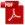### 7.3.3. Axial Spring Design

Reference: Abbott, Richard. Analysis and Design of Composite and Metallic Flight Vehicle Structures 3 Edition, 2019

7.3.3.1. Belleville Washer Design

This method is taken from  (NASA TM X-73305, 1975) and is a relatively simple method.

Belleville springs are used where space requirements dictate a short range of motion.

The design depends on the following parameters:

M, C1 and C2 are constants which can be calculated from the formulas below:

The deflection load formula using these constants is:

The stress formula is as follows:

In the stress formula, it is possible for the term

to become negative when δ is large.

When this occurs the term inside the bracket should be changed to read

Note that the load deflection curve for Belleville washers is not linear.

This is shown in Figure 7.3.3‑1.

Belleville washers can be stacked to obtain the desired load-deflection characteristics; the accepted methods are shown in the figure below:

As the number of washers increases so does the friction in the stacks. This may not be uniform and can result in springs which are erratic and difficult to predict.

### 7.3.3. Axial Spring Design

Reference: Abbott, Richard. Analysis and Design of Composite and Metallic Flight Vehicle Structures 3 Edition, 2019

7.3.3.1. Belleville Washer Design

This method is taken from  (NASA TM X-73305, 1975) and is a relatively simple method.

Belleville springs are used where space requirements dictate a short range of motion.

The design depends on the following parameters:

M, C1 and C2 are constants which can be calculated from the formulas below:

The deflection load formula using these constants is:

The stress formula is as follows:

In the stress formula, it is possible for the term

to become negative when δ is large.

When this occurs the term inside the bracket should be changed to read

Note that the load deflection curve for Belleville washers is not linear.

This is shown in Figure 7.3.3‑1.